# Inductive dimension

(diff) ← Older revision | Latest revision (diff) | Newer revision → (diff)
large inductive dimension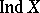, small inductive dimensionDimension invariants (cf. Dimension invariant) of a topological space; both are defined by means of the notion of a partition between two sets. The definition is by induction, as follows. For the empty space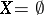one sets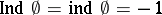. Under the hypothesis that all spacesfor whichare known, whereis a non-negative integer, one puts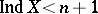if for any two disjoint closed subsetsandofthere is a partitionbetween them for which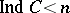. Here, a closed setis called a partition betweenandinif the open set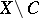is the sum of two open disjoint sets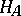andcontainingand, respectively. This definition transfers to the definition of small inductive dimension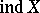by taking one of the setsorto consist of a single point, while the other is an arbitrary closed set not containing this point.
The large inductive dimension was defined for a fairly wide class of (metric) spaces by L.E.J. Brouwer . The small inductive dimension was defined independently by P.S. Urysohn  and K. Menger . The study of inductive dimensions and, more generally, of dimension invariants, is only of interest under the hypothesis that the spacesatisfies sufficiently strong separation axioms, mainly the axiom of normality (cf. Separation axiom).